# Multi-Class Text Classification with FastAi along with built models

## Predicting different gender classes based on tweets(text) data by applying NLP, deep learning concepts and Machine Learning models

The code is available here in the repository.

Classification problems are now a days very common in the field of Data Science for solving Machine Learning tasks, NLP(Natural Language Processing) problems. Classification generally predicts categorical class labels based on the training set and the values (class labels) in classifying attributes and uses it in classifying new data.

After I have got my hands full on my first Capstone project in Springboard Data Science Career Track, I have moved on to my second project. I wanted to work on something more in depth in the Machine Learning field. So, I chose working with the text/tweets data along with image classification of the genders, which I was very much interested in. NLP is used and its different methods pave the way for achieving a solution for performing the analysis. and classification.

For this project, I chose a Kaggle problem and the dataset needed to get me through with this gender classification. We can explore how multi-classification works.

# 1. DEFINING THE PROBLEM

The main challenge of this project is to view a Twitter profile as well as take the text, description features from this dataset, and predict whether the user was a male, a female, or a brand (non-individual). This is a multi-classification problem which can be explored through NLP using deep learning library such as “fastai” as one of the techniques. Apart from that, Machine Learning models were also built from scratch to compare how it performs with the concept of Transfer Learning from the deep learning library.

• Different types of questions can be answered from the analysis which will be discussed in the next section, like

a) How well do words in tweets and profiles predict user gender

b) What are the words that strongly predict male or female gender

# 2. DATASET

The dataset contains of 20050 rows and 26 columns/features each with a username, a random tweet, account profile and image, location, and even link and sidebar color. Twitter User Gender Classification . This data set was used to train a CrowdFlower AI gender predictor. Also, this dataset contains profileImages as image url’s, which is very useful for Image Classification to detect the gender.

a. Data Cleaning

So, this part of cleaning the data was not bad. I had many values of unknown in the gender column. So, I had to drop them since our target variable to is to predict the gender, and we cannot keep the information which we do not have any clue about, and that it does not carry much information too.

I also dropped unnecessary columns like ‘gender_gold’, ‘trusted_judgements’ and other features which were not useful in determining the gender. There were missing values in the description feature, which will be very useful in text analysis and predictive modelling, in later sections. So, I combined description with the text feature in order to compensate for the missing values.

# 3. DATA PREPROCESSING

In this step, I applied different techniques of analysis from the obtained text and description columns, since in Natural Language Processing, a lot of information can be analyzed from it.

a. Regex to clean unnecessary characters

b. Tokenization

c. Stopwords

d. Lemmatization

Lemmatization, Tokenization and stop words removal are used to reduce the number of words by either removing common words with no significant content (stopwords such as and, or, if ecc), or to extract the core of the different words and account them for one (eg. playing, played plays → play).

Here, I created different functions for cleaning the tweets(combination of text and description) feature, creating more useful meaning for different documents. Below, I added my code on how I have approached.

a. Regular Expressions(Regex):

`import re# function for # regex to clean unnecessary charsdef cleaning_text(text):     # remove everything except alphabets and also @,#    text = re.sub("[^a-zA-Z]"," ",text)     text = re.sub('[!@#\$_]', '', text)    text = text.replace("co","")    text = text.replace("http","")    # remove whitespaces     text = ' '.join(text.split())     # convert text to lowercase     text = text.lower()     return text#Apply the above cleaning function to the Tweets columnsub_df['Tweets_cleaned'] = sub_df['Tweets'].apply(lambda x: cleaning_text(x))`

b. Tokenization:

It is the process of breaking a stream of text up into words, phrases, symbols, or other meaningful elements called tokens.This is useful for further processing such as text-mining, where these tokens serves as an input.

`from nltk.tokenize import word_tokenize# function to apply tokenization to the Tweets cleaned columndef tokenize(text):    token_words= word_tokenize(str(text))    return " ".join(token_words)sub_df['Tweets_cleaned_tokenized'] = sub_df['Tweets_cleaned'].apply(lambda x: tokenize(x))`

c. Stop-Words:

We can remove the stop-words from the nltk library. Stop words are a set of commonly used words in any language. Why removing stop words are critical to many applications? If we remove the words that are very commonly used in a given language, we can focus on the important words instead that has more weight.

`from nltk.corpus import stopwords# function to apply stopwords to the Tweets cleaned tokenized columndef stopwords_clean(text):    stop_words = set(stopwords.words('english'))    no_stopword_text = [w for w in str(text).split() if not w in stop_words]    return " ".join(no_stopword_text)sub_df['Tweets_cleaned_nostop'] = sub_df['Tweets_cleaned_tokenized'].apply(lambda x: stopwords_clean(x))`

d. Lemmatization:

It is a technique of text normalization . In lemmatization, the words are replaced by the root words(lemma) or the words with similar context.

`import nltkfrom nltk.stem import WordNetLemmatizerlemma= WordNetLemmatizer()# function for lemmatizing wordsdef lemmatize_text(text):    lemma_text = [lemma.lemmatize(word) for word in text]    return "".join(lemma_text)sub_df['Tweets_cleaned_lemmatized'] = sub_df['Tweets_cleaned_nostop'].apply(lambda x: lemmatize_text(x))`

# 4. EXPLORATORY DATA ANALYSIS

Exploratory analysis is one of the important steps in analyzing the data properly. Mainly, this is useful in discovering the patterns and anomalies in the data, through statistical tests and visual explanations.

After having my Tweets feature cleaned using the important techniques described above, it is time for me to analyze on how the text data can be well explored.

The bar plot above depicts the counts of frequently used words in a tweet(combination of text and description features). This gives us a great insight on how important/words weigh.

I have also used another visualization technique called Word Cloud. It represents the frequency or the importance of each word. The bigger the word is, the more importance it weighs in. Below is the code I used to generate it. There are no duplicates in it, since I have used a small snippet of Python code to remove them as well.

`# Generating a word cloud of frequency of textfrom wordcloud import WordCloudwordcloud = WordCloud(background_color="white", width=1500, height=1000).generate(' '.join(sub_df['Tweets_cleaned_lemmatized']))plt.imshow(wordcloud, interpolation='bilinear')plt.axis("off")plt.show()`

# 5. INFERENTIAL STATISTICS: 2 TAILED T-TEST?

Here, there was something interesting which I wanted to test to make sure that the tweets(text) data coming out from both gender had an identical average length of the words in the data. This was my Null Hypothesis (H0). So, I wanted to perform a 2 tailed t-test. A two tailed test allows us to find the area in the middle of a distribution. Usually, every hypothesis test assumes the plot to be normally distributed.

The t-test for the non cleaned version of the text data was performed and obtained the following result. Here the p-value was almost 0(I would say 0). So I could reject the null hypothesis and say that there was indeed a statistically significant difference between the gender’s average length of words in a text.

# 6. PREPARING THE DATA FOR MODELING

## i. BAG-OF-WORDS :

Now that we have analyzed the text data, the next step in the process is to properly convert/transform the text data into Machine Learning understanding algorithms. We cannot directly feed our text into that algorithm. Since our predictor variable here is text, how can we convert the data that is suited for the algorithms? There is a technique called Bag-Of-Words(BOW) . The bag-of-words model is simple to understand and implement. It is a way of extracting features from the text for use in machine learning models.

Here, I used CountVectorizer to convert a collection of text documents to a matrix of token counts. This is performed on the predictor/independent feature/variable(X), which is the text(Tweets) data. It counts the term frequencies, which means counting the occurrences of tokens and building a sparse matrix of documents of the tokens in the dataset.

## ii. LABEL-ENCODING:

The predictor variable has been converted to a suitable format for my model. But the target variable(y) has the classes available, which is Categorical in nature. So, I had to convert the categorical text data into model understandable numerical data. Hence, I used LabelEncoder class here. Below is a code snippet of how it is used. We import LabelEncoder from sklearn and then fit-transform the data, for it to get encoded.

`from sklearn.preprocessing import LabelEncoderencoder = LabelEncoder()y = encoder.fit_transform(y)`

# 7. BUILDING MODELS

Now that the text data has been prepared and preprocessed, I would now build a classifier algorithm/model to classify the predictions of different genders(Male, female and brand). Since there are 3 labels of classes, this is a multi-class classification. I use the Tweets(non_cleaned_version) of the file to predict the gender from the text. Later, I will also show the results of cleaned version of the text data. The Training and Testing data have been split into 70% and 30% respectively using Machine Learning ‘sci-kit’ library.

`from sklearn.model_selection import train_test_splitX_train, X_test, y_train, y_test = train_test_split(X, y, test_size=0.3, random_state=12, stratify=y)`

## a. LOGISTIC REGRESSION:

Logistic regression is a classification algorithm used to assign observations to a discrete set of classes. It is a special case of linear regression where the target variable is categorical in nature. The hypothesis of logistic regression tends it to limit the cost function between 0 and 1. Here, in this case, it is a Multinomial Logistic Regression, since we have 3 different classes of gender.

Using Pipeline:

I have used Machine Learning Pipeline. Pipeline is very useful for automating the Machine Learning workflow. Also, I set the parameter grid for selecting the best parameters using GridSearchCV of 5 folds for my Logistic Regression Model(penalty = ‘l1’, C= 0.1). It also contains cross validation within the Gridsearch on the dataset. This method is called hyperparameter tuning, where optimization is the key factor for selecting the best parameters.

The below figure shows the results for non-cleaned version of text data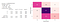Fig 9: Classification Report and Confusion Matrix for Logistic Regression of non cleaned text data

The below figure shows the results for cleaned version of text data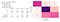Fig 10: Classification Report and Confusion Matrix for Logistic Regression of cleaned text data

I have obtained the above using Label Encoder’s inverse_transform class, to decode the classes encoded by the Encoder.

## b. RANDOM FOREST CLASSIFIER

I have used Random Forest ensemble method which is a non linear model. In this, I use a Classifier, since my output contains multiple classes to determine. It uses multiple decision trees and a technique called as bagging. This combines multiple decision trees in determining the final output rather than relying on individual decision trees. By averaging several trees, there is a significantly lower risk of overfitting. I have used GridsearchCV of 5 folds as well to find the best parameters for my dataset(n_estimators= 50, max_depth=15).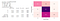Fig 11: Classification Report and Confusion Matrix for Random Forest Classifier of non cleaned text data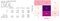Fig 12: Classification Report and Confusion Matrix for Random Forest Classifier of cleaned text data

## c. SVM(SUPPORT VECTOR MACHINE):

This is a C-Support Vector Classification. SVM’s are mainly used for the below points.

• SVM maximizes margin, so the model is slightly more robust (compared to linear regression), but more importantly: SVM supports kernels, so it can be modelled using non linear points too.
• These are effective in high dimensional spaces.
• Still effective in cases where number of dimensions is greater than the number of samples.
• Uses a subset of training points in the decision function (called support vectors), so it is also memory efficient.

Here I have also selected the best parameters using GridSearchCV of 5 folds (C=1,gamma=’scale’, kernel=’linear’).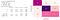Fig 13: Classification Report and Confusion Matrix for SVM of non cleaned text data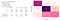Fig 14: Classification Report and Confusion Matrix for SVM of cleaned text data

METRICS USED:

1. Classification Report : The classification report displays the precision, recall, F1, and support scores for the model. It builds a text report showing the main classification metrics.

a. Precision = TP/(TP + FP) : Accuracy of positive predictions.

b. Recall = TP/(TP+FN) : Fraction of positives that were correctly identified.

c. F1-Score = 2 * (precision * recall) / (precision + recall): It is a harmonic mean of the precision and recall, where an F1 score reaches its best value at 1 and worst score at 0.

d: Support: It is the number of occurrences of the given class in the dataset.

2. Confusion Matrix: It is a performance measurement for machine learning classification problem where output can be multiple classes. It is a table with different combinations of predicted and actual values. Having False Positives(FP) is considered a Type-I error, where the False Negatives(FN) is a Type-II error. Typically, Type-I error should be as less as possible(ideally, none at all).

# 8. FAST-AI

Fastai is a deep learning Library, which I have used as one of the options to train my model on. I would not say I have trained from scratch, because the fastai library operates on the method called Transfer Learning. It is a technique where instead of training a model from scratch, we reuse a pre-trained model and then fine-tune it for another related task. It consists of a pre-trained model where I can use my dataset to classify the gender on the text data. It is a very useful technique which makes the classification pretty accurate

Here, I have used Google Colab notebook for my fastai library for classification. Colab is a free Jupyter notebook environment that runs entirely in the cloud. Most importantly, it does not require a setup. Colab supports many popular machine learning libraries which can be easily loaded in your notebook.

Here, I have used the method `from_df` of the `TextLMDataBunch` to create a language model specific data bunch. The necessary data preprocessing happens behind the scenes. Language Model learner is used. It is used to predict the probability of a sequence of words. A nice feature in a language model is that it is generative, which means that it aims to predict the next word given a previous sequence of words. But here, in our case, it is trained on our dataset used to classify the correct gender using the text data.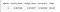Fig 16: Accuracy of a Language model trained on 1 epoch

We can already see that by training the Language model on our Dataset for 1 epoch, it already has obtained an accuracy of 32%.

Also, I used TextClasDataBunch to get the data ready for a text classifier. We now use the data_clas object we created earlier to build a classifier with our fine-tuned encoder. The learner object can be done in a single line.

## How to train the model?

To train our model, the fastai library provides important classes needed for this. Here, I used fit_one_cycle.

`fit_one_cycle()` uses huge learning rates to train models significantly quicker and with higher accuracy.

Now, we can also continue training the model to try and minimize both the training and validation loss to as minimum as possible. We use the concept of freeze_to and unfreeze.

Freeze(freeze_to) and unfreezing(unfreeze) is helpful in us deciding which specific layers of the model we want to train at a certain point of time in an epoch.

For improving the accuracy further, I used freeze_to method with different layers in it. Trained last two layers using freeze_to(-2), train it a little bit more. unfreeze the next layer freeze_to(-3), train it a little bit more. unfreeze() the whole thing. It is better to first train few layers and then unfreeze to train the entire model on the dataset. It took 5 epochs to train the model for achieving that particular accuracy.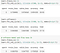Fig 17: Freezing and unfreezing the model to train the layers without changing the weights during training

There is a parameter here, which is moms=(0.8.0.7) which describes momentum. While training recurrent neural networks (RNNs), this helps to decrease the momentum for a bit.

Here it is important to note that we are checking for the trend of error vs. epochs. I could have easily tried to fine tune the model more, but the training loss started decreasing after the above results, which could have caused data to overfit, so I have stopped training the model.

I have also plotted the confusion matrix. It is a good technique to summarize the performance of a classification algorithm. We use ClassificationInterpretationclass here.

# 9. RESULTS

As we can see from the above, even though we built our models from scratch and trained them on the whole text, we get good accuracy scores. But in comparison, we used a transfer learning technique using fastai library and we are able to get a great increase in the results in classifying different genders(Below figures shows the results). So, that is a pretty powerful technique to implement later when we can add a few more features along with text, since we can decrease the training and validation loss too, which is important for neither overfitting nor underfitting the data.

Apart from this, I believe that the models which I built, some of them are highly interpretable(to which extent can we consistently predict the model’s result) like Logistic Regression like probabilistically, SVM for high accuracy, and Random Forests with a good amount of interpretation and accuracy as well.

## a. Comparison of model performances: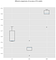Fig 20: Comparison of ML models → Left figure is non cleaned version of text data ; Right figure is cleaned version of text data(K-Fold =5)

# 10. FUTURE WORK

There was a lot which I got to work on in this project, where I had to predict the gender based on text data. I have used fastai deep learning library to correctly identify the gender with the pre-trained models.

• In this dataset, I tried to use Images as profile-Image for classification as well, but I did not have the chance to work on it. Definitely, this can drastically improve on the work and would be an interesting alternative problem to tackle along with what I have been doing.
• Apart from this, I can incorporate some more features along with the text data into my predictors, so I can see some improvement on the classification.
• With the models, SVM can be worked along with PCA for reducing the feature space, where I encode my text data as separate vectors.
• It takes time to execute SVM alone. Probably adding dimensionality reduction can work.

I have enjoyed working on this project of NLP. It was very interesting to learn different concepts in fastai, a deep learning library. This would not have been possible without the feedback from my Springboard mentor, Konstantin Palagachev, who has helped me in every step of the way.

Feel free to reach out to me on LinkedIn if you have any questions regarding this project. Thanks!